Scripting

# How to Plot a Function in PythonThe matplotlib.pyplot module’s plot() method offers a single interface for constructing many sorts of graphs. This function accepts any two arguments, in our case, x and y, and generates a visual representation of the relevant points in x and y. Following these procedures, we may plot a function:

1. First of all, we import the pyplot function from the matplotlib module.
2. Then we define values for the x and y variables, which are dependent on the type of function to be plotted.
3. Then we use the plt.plot() function to draw the figure.
4. Lastly, we use the plt.show() function to display the plot.

Let’s consider the following examples for plotting functions.

## Example 1: Identity function

```From matplotlib, import pyplot as plt.

x = [0, 1, 2, 3, 4, 5]

y=x

plt.plot(x, y)

plt.show()```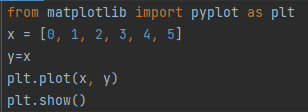Figure 1: Identity Function

Output: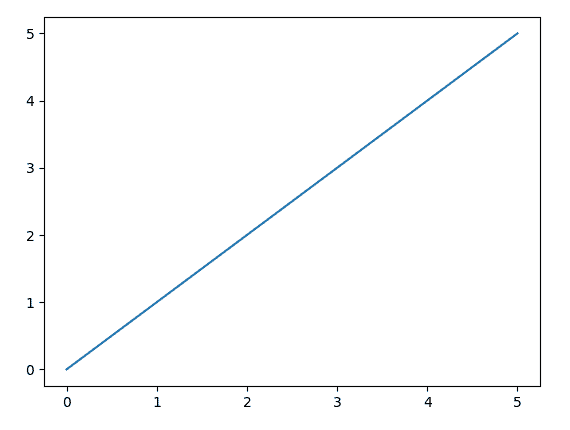In an identical function, values of x and y are the same, so we get a straight line plot as shown in figure 2.

## Example 2: Sine function

```from matplotlib import pyplot as plt

import numpy as np

x = np.linspace(0, 10, 50)

plt.plot(x, np.sin(x))

plt.show()```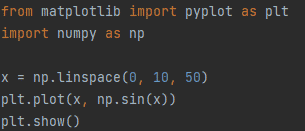Output: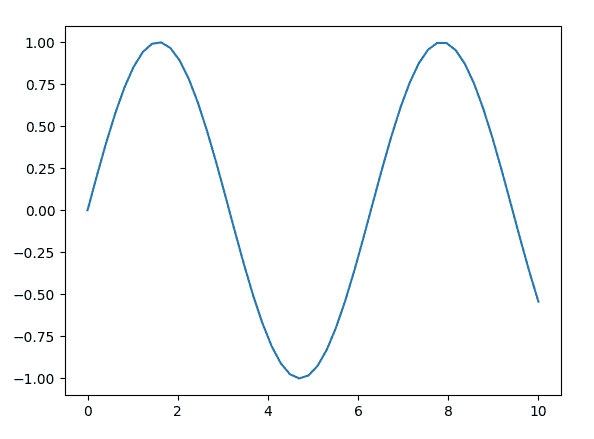In example 2, we are using the NumPy module. As x is generated using the np.linespace function, which returns evenly spaced numbers between 0 and 1 over a specified interval. Here, y is also the sine function of the NumPy module. The plot for the sine function is shown in figure 4.

```from matplotlib import pyplot as plt

import numpy as np

x =np.array([1, 2, 3, 4, 5, 6, 7, 8, 9, 10])

a = 3

b = 8

c = 9

y = a * (x ** 2) + b * x + c

print('Values of x: ', x)

print('Values of y: ', y)

plt.plot(x, y)

plt.xlabel("Values of x")

plt.ylabel("Values of y")

plt.show()```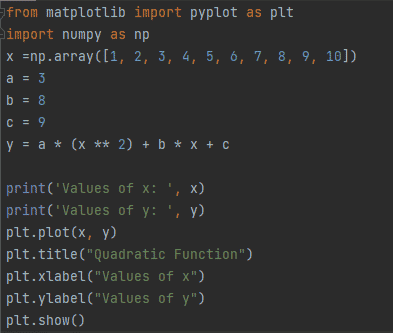Output: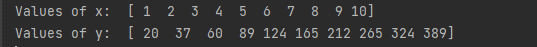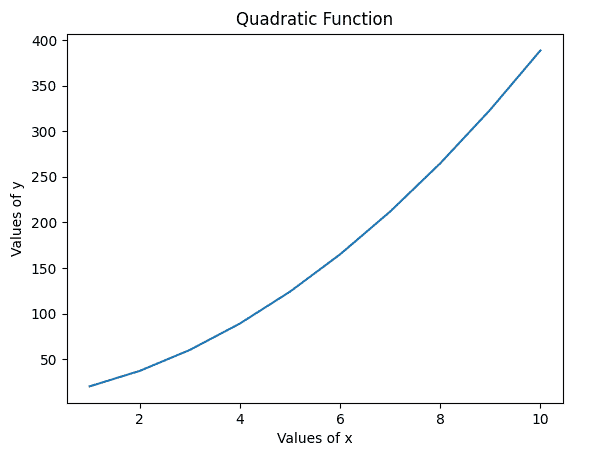In example 3, we have an x variable list which is converted to a matrix using the array function of the numpy module. The value of y is calculated by inserting values of variables and constants into a quadratic equation. The output of the quadratic function is shown in figure 6. A plot is drawn for quadratic function as shown in figure 7.

## Example 4: Cubic Function

```from matplotlib import pyplot as plt

import numpy as np

x =np.array([-4, -3, -2, -1, 0, 1, 2, 3, 4])

a = 2

b = 4

c = 6

d = 8

y = a * (x ** 3) + b * (x ** 2) + c * x + d

print('Values of x: ', x)

print('Values of y: ', y)

plt.plot(x, y)

plt.xlabel("Values of x")

plt.ylabel("Values of y")

plt.title("Cubic Function")

plt.show()```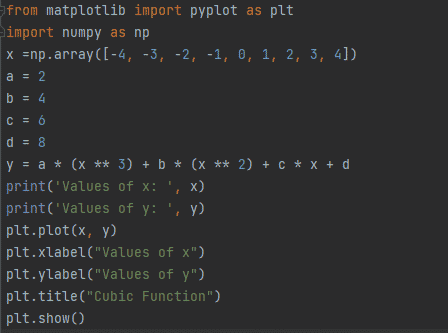Output: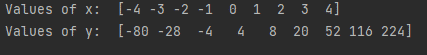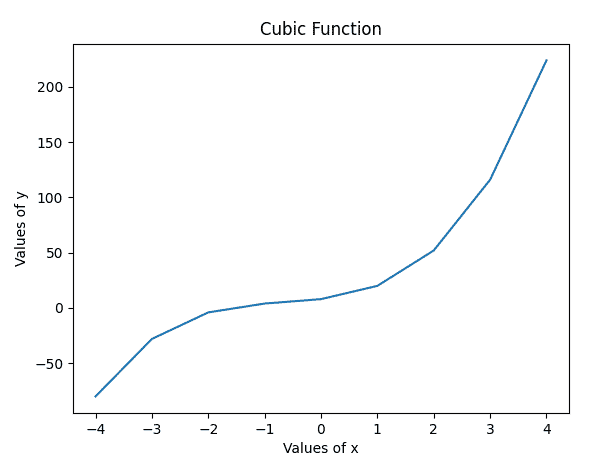In example 4, y represents the cubic equation. Values for cubic functions are calculated and displayed in figure 9. A cubic function plot is drawn in figure 10.